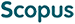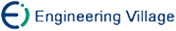Pan Shijie, Gao Fei, Wan Linchun, Qin Sujuan, Wen Qiaoyan. Quantum Algorithm for Spectral Regression[J]. Journal of Computer Research and Development, 2021, 58(9): 1835-1842. DOI: 10.7544/issn1000-1239.2021.20210366
 Citation: Pan Shijie, Gao Fei, Wan Linchun, Qin Sujuan, Wen Qiaoyan. Quantum Algorithm for Spectral Regression[J]. Journal of Computer Research and Development, 2021, 58(9): 1835-1842. DOI: 10.7544/issn1000-1239.2021.20210366# Quantum Algorithm for Spectral Regression• Subspace learning is an important research direction in the field of machine learning. In order to reduce the complexity of subspace learning, Cai et al. proposed a spectral regression dimensionality reduction framework, and proposed efficient spectral regression for subspace learning that constructed their graph by incorporating the label information. In recent years, the development of quantum computing has made it possible to further reduce the complexity of subspace learning algorithms. Meng et al. pioneered the quantum algorithm for spectral regression (MYXZ algorithm). In this article, the limitations of the MYXZ algorithm are pointed out. We point out that MYXZ algorithm uses sparse Hamiltonian simulation technology to process the matrix generated by the weight matrix, but this matrix is a dense matrix in some cases. In response to this situation, we propose an improved quantum spectral regression algorithm. Our improved algorithm uses quantum singular value estimation technology, which has a polynomial acceleration compared with MYXZ algorithm when dealing with dense matrices. In addition, we propose a new quantum algorithm to accelerate the classical efficient spectral regression. The problems that our new algorithm can handle cannot be handled by MYXZ algorithm. Our algorithm utilizes quantum ridge regression and quantum matrix vector multiplication technology. Under the same parameter conditions, our algorithm has polynomial acceleration effect compared with the classical algorithm.
•Turn off MathJax
Article Contents/DownLoad:  Full-Size Img  PowerPoint